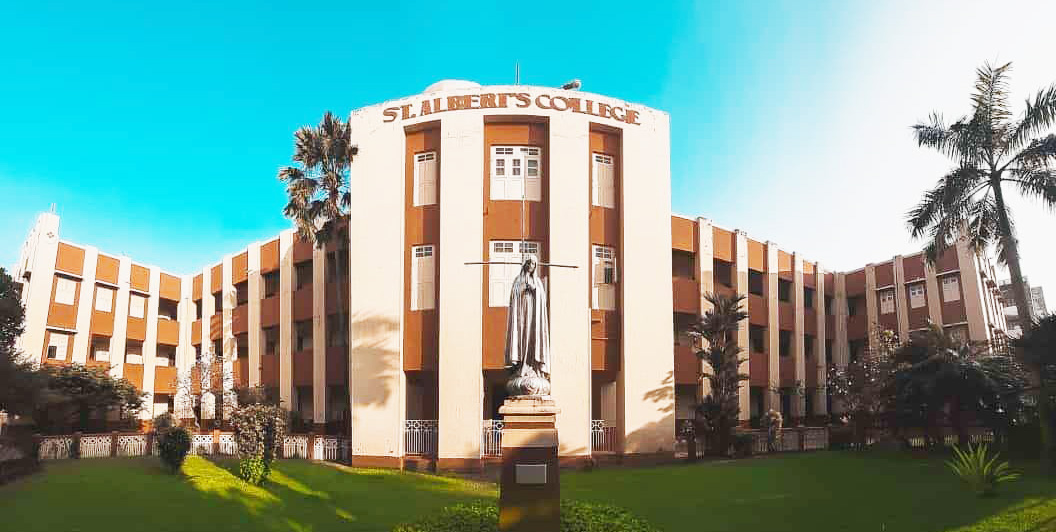# Probability And StatisticsSubitha Sudheer
Computer Science
₹1,000.00• 22 students
• 5 lessons
• 0 quizzes
• 0 week duration
22 students

Probability And Statistics is a Complementary course for third semester B.Sc  Computer Science students. The course consists five modules . The course starts with an introduction about Statistics and the first module compresses the same content  that is history, meaning ,different definitions of Statistics, data collection,  methods etc. From the second Module the core section is starting that is about Probability. In shortly the course enables the students to handle various Statistical methods along with the applications of Probability

Syllabus is as follows

• ##### Module 1-Introduction to Statistics, Basic concepts.

This particular module cover the introduction and basic concepts of Statistics. Main topics of the module are as follows.Introduction to Statistics, Basic concepts, population and sample, Collection of data, census and sampling .Methods of sampling-Random and Non Random sampling methods. Frequency distributions-Measures of central tendency, measures of dispersion, moments, skewness and kurtosis. Correlation and Regression analysis-Types of correlations, Methods of studyng simple correlation, properties of correlation coefficient.

• ##### Module 2-Different approaches to probability
No items in this section
• ##### Module 3 - Expectation and its properties
No items in this section
• ##### Module 4 -Sampling distributions
No items in this section0.00 average based on 0 ratings

5 Star
0%
4 Star
0%
3 Star
0%
2 Star
0%
1 Star
0%
₹1,000.00